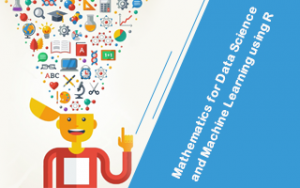# Mathematics for Data Science and Machine Learning using R

Learn the fundamental mathematics for Data Science, AI &ML using R

Language - English Published on 10/2019

#### Description

Without a doubt, data science has become one of the most important fields in the current world. From banking to healthcare to business, it is essential for everyone and that is the main reason behind the significant increase in the demand of data science expert throughout the world. Considering all these things, we have designed this online course which teaches you all mathematics of data science including R programming language. R programming language was created especially for data analysis, performing statistics and graphical representation in a better way.

# What makes this online tutorial unique?

Though several tutorials on data science are already available, this online course is one of its kind guide having hand-picked topics revolving around data science. It includes some of the essential concepts of foundational mathematics for data science using R programming language. You will explore numerous sections with over 9 hours of video content lectured by one of the world’s veteran statistician and data scientists, Timothy Young. He explains all the concepts in the most simplest way possible for easy and effective learning of mathematics behind data science.

# This course includes -

• Introduction- Data Science, Machine Learning & R Programming Language
• Linear Algebra- Scalars, Vectors & Metrices
• Section Calculus- Function & tangent Lines, Derivatives
• Vector Calculus -
• Orthogonal Vectors and Linear Independence
• Eigenvectors and Eigenvalues
• Vectors Gradient Descent, and so much more!

It is clear that data science is becoming important for both developers as well as industries causing a significant increase in the demand of the experts. Like several others, if you are the one who is facing difficulties with all the mathematical concepts for data science then this is the right time and this online tutorial is the right course designed for exclusively for you!

#### What Will I Get ?

• Master the fundamental mathematical concepts required for Datas Science and Machine Learning
• Learn to implement mathematical concepts using R
• Master Linear alzebra, Calculus and Vector calculus from ground up
• Master R programming langauge

#### Requirements

• Basic knowledge of Statistics and Mathematics is required to complete the course

#### Feedbacks (0)

• No Feedbacks Posted Yet..!This Course Includes :

10.5 hours

64 Lectures

6 Resources